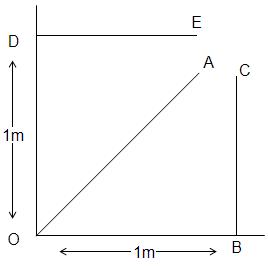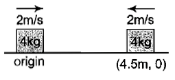A particle of mass m moves with a constant velocity along 3 different paths DE, OA & BC. Which of the following statements is not correct about its angular momentum about point O?(A) It is zero when it is at A and moving along OA.

(B) The same at all points along the line DE.

(C) Of the same magnitude but oppositely directed at B and  D.

(D) Increases as it moves along the line BC.

Concept Questions :-

Angular momentum
High Yielding Test Series + Question Bank - NEET 2020

Difficulty Level:

High Yielding Test Series + Question Bank - NEET 2020

Difficulty Level:

A sphere is rolling down a plane of inclination $\theta$ to the horizontal.  The acceleration of its centre down the plane is

(1) g sin $\theta$

(2) less than g sin $\theta$

(3) greater than g sin $\theta$

(4) zero

High Yielding Test Series + Question Bank - NEET 2020

Difficulty Level:

A body at rest breaks into two pieces of equal masses.  The parts will move

(1) in same direction

(2) along different lines

(3) in opposite directions with equal speeds

(4) in opposite directions with unequal speeds

High Yielding Test Series + Question Bank - NEET 2020

Difficulty Level:

A bob of mass m attached to an inextensible string of length l is suspended from verticle support.  The bob rotates in a horizontal circle with an angular speed  about the vertical.  About the point of suspension.

(1) Angular momentum is conserved

(2) Angular momentum changes in magnitude but not in the direction

(3) Angular momentum changes in direction but not in magnitude

(4) Angular momentum changes both in direction and magnitude

High Yielding Test Series + Question Bank - NEET 2020

Difficulty Level:

In free space, a rifle of mass M shoots a bullet of mass m at a stationary block of mass M at a distance D away from it. When the bullet has moved through a distance d towards the block, the centre of mass of the bullet - block system is at a distance of

(1) $\frac{\left(D-d\right)}{M+m}$ from the bullet

(2) $\frac{md+MD}{M+m}$ from the block

(3) $\frac{2md+MD}{M+m}$ from the block

(4) $\frac{\left(D-d\right)M}{M+m}$ from the bullet

Concept Questions :-

Center of mass
High Yielding Test Series + Question Bank - NEET 2020

Difficulty Level:

Blocks A and B are resting on a smooth horizontal surface given equal speeds of 2 m/s in the opposite sense as shown in the figure.At t = 0, the position of blocks are shown, then the coordinates of centre of mass at t = 3s will be

1.  (1, 0)

2.  (3, 0)

3.  (5, 0)

4.  (2.25, 0)

Concept Questions :-

Center of mass
High Yielding Test Series + Question Bank - NEET 2020

Difficulty Level:

A wheel is at rest. Its angular velocity increases uniformly and becomes 80 rad/s after 5 s. The total angular displacement is

Concept Questions :-

Rotation motion : Introduction
High Yielding Test Series + Question Bank - NEET 2020

Difficulty Level:

A force $-\mathrm{F}\stackrel{^}{\mathrm{k}}$ acts on O, the origin of the coordinate system. The torque about the point (1, -1) is :

1. $-\mathrm{F}\left(\stackrel{^}{\mathrm{i}}+\stackrel{^}{\mathrm{j}}\right)$

2. $\mathrm{F}\left(\stackrel{^}{\mathrm{i}}+\stackrel{^}{\mathrm{j}}\right)$

3. $-\mathrm{F}\left(\stackrel{^}{\mathrm{i}}-\stackrel{^}{\mathrm{j}}\right)$

4. $\mathrm{F}\left(\stackrel{^}{\mathrm{i}}-\stackrel{^}{\mathrm{j}}\right)$

Concept Questions :-

Torque
High Yielding Test Series + Question Bank - NEET 2020

Difficulty Level:

Moment of inertia of an object does not depend upon

1.  mass of object

2.  mass distribution

3.  angular velocity

4.  axis of rotation

Concept Questions :-

Moment of inertia